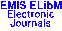Bulletin, Classe des Sciences Mathématiques et Naturelles, Sciences mathématiques naturelles / sciences mathematiques Vol. CXXXIX, No. 34, pp. 1–16 (2009)

Next Article

Contents of this Issue

Other Issues

ELibM Journals

ELibM Home

EMIS Home

Pick a mirror

## Note on Estrada and $L$-Estrada indices of graphs

### G. H. Fath–Tabar, A. R. Ashrafi and I. Gutman

Department of Mathematics, Faculty of Science, University of Kashan, Kashan 87317–51167, I. R. Iran
Faculty of Science, University of Kragujevac, P. O. Box 60, 34000 Kragujevac, Serbia

Abstract: Let $G$ be a graph of order $n$ . Let $\lambda_1,\lambda_2,\ldots,\lambda_n$ be its eigenvalues and $\mu_1,\mu_2,\ldots,\mu_n$ its Laplacian eigenvalues. The Estrada index $EE$ of the graph $G$ is defined as the sum of the terms $e^{\lambda_i} , i=1,2,\ldots,n$ . In this paper the notion of Laplacian–Estrada index ($L$-Estrada index, $LEE$) of a graph is introduced. It is defined as the sum of the terms $e^{\mu_i} , i=1,2,\ldots,n$ . The basic properties of $LEE$ are established, and compared with the analogous properties of $EE$ . In addition, the Estrada and $L$-Estrada indices of some important classes of graphs are computed.

Keywords: Spectrum (of graph), Laplacian spectrum (of graph), Estrada index, Laplacian–Estrada index

Classification (MSC2000): 05C50

Full text of the article: (for faster download, first choose a mirror)